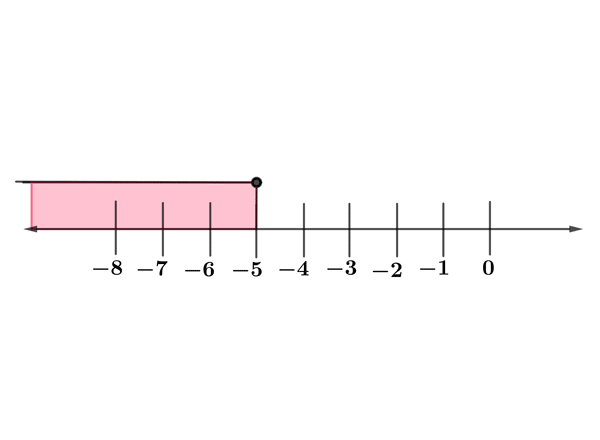Math Calculators, Lessons and Formulas

It is time to solve your math problem

mathportal.org
• Linear inequalities
• Solving linear equations and inequalities
• Solving elementary inequalities

# Solving elementary inequalities

ans:
syntax error
C
DEL
ANS
±
(
)
÷
×
7
8
9
4
5
6
+
1
2
3
=
0
.
auto next question
calculator
•  Question 1: 1 pts Solve inequality $x-7>2.$
 $x>-5$ $x>9$ $x<9$ $x<-5$
•  Question 2: 1 pts Solve inequality $x+ \dfrac{2}{3}<2.$
 $x> \dfrac{4}{3}$ $x>\dfrac{2}{3}$ $x< \dfrac{2}{3}$ $x< \dfrac{4}{3}$
•  Question 3: 1 pts Solve inequality $-3x+5\leq 26.$
•  Question 4: 1 pts The solution of inequalities $-3a+7\leq 4$ is $a\leq 1.$
•  Question 5: 2 pts Give the inequality which produces the range shown in the figure.$x<-5$ $x>-5$ $x\leq -5$ $x\geq -5$
•  Question 6: 2 pts Solve inequality $3x-6-8x<44.$
 $x<-10$ $x>-10$ $x\geq -10$ $x\leq -10$
•  Question 7: 2 pts Solve inequality $-4x+9\geq x-21.$
•  Question 8: 2 pts Solve inequality $2x+3>-6+2x.$
•  Question 9: 3 pts Solve inequality $3x-\dfrac{4}{5}\geq -x$
 $x<\dfrac{1}{5}$ $x> \dfrac{1}{5}$ $x\geq \dfrac{1}{5}$ $x\leq \dfrac{1}{5}$
•  Question 10: 3 pts Solve inequality $-0.12x+3.4<0.84+5x$
 $x>\frac{1}{2}$ $x<\frac{1}{2}$ $x>\frac{1}{5}$ $x<\frac{1}{5}$
•  Question 11: 3 pts Solve inequality $-0.5x-3.69 < x-1.9-2.39$
 $x>-0.4$ $x<0.4$ $x>0.4$ none of these
•  Question 12: 3 pts Solve inequality $1\leq 7+2x \leq 9$
 $x \in [-3,1]$ $x \in (-3,1]$ $x \in [-3,1)$ $x \in (-3,1)$